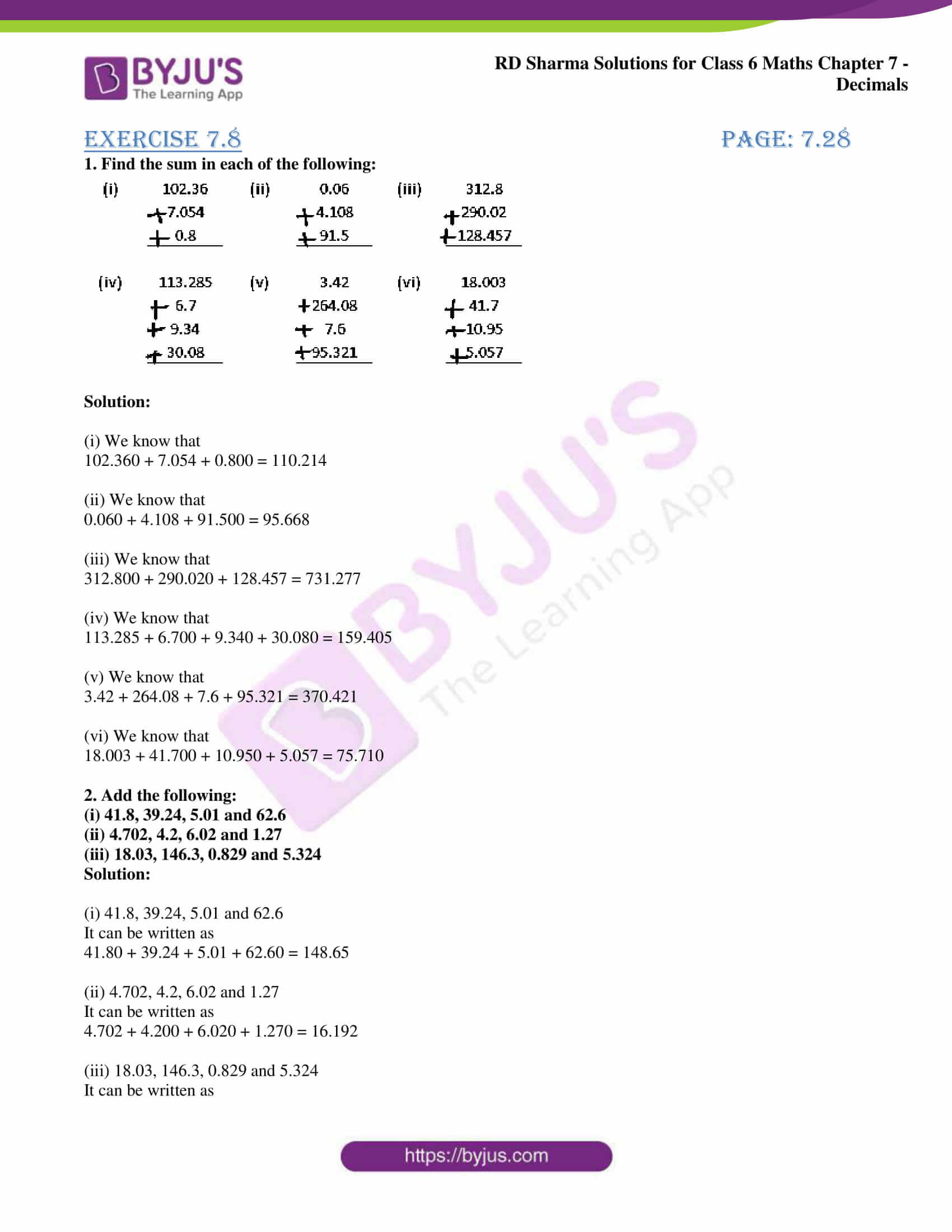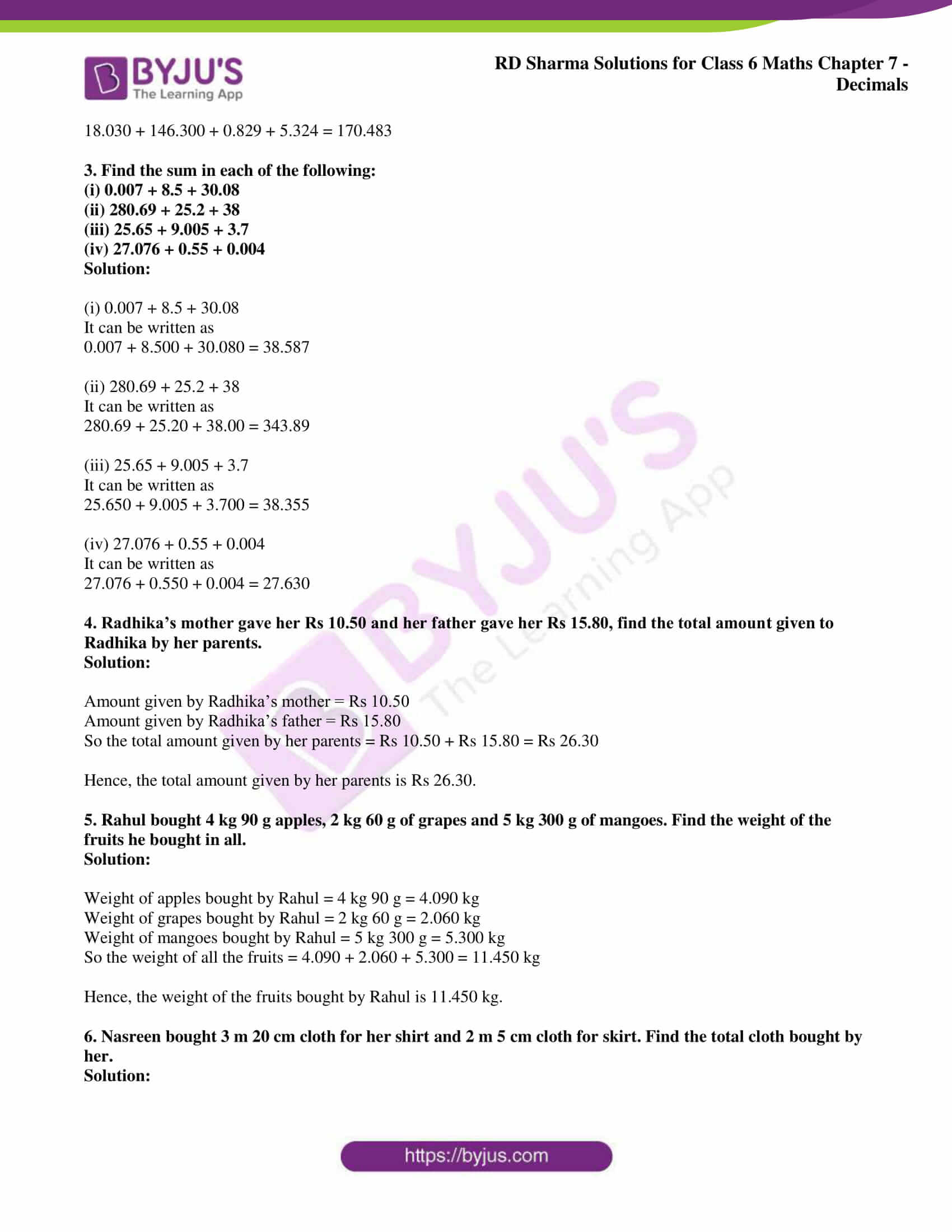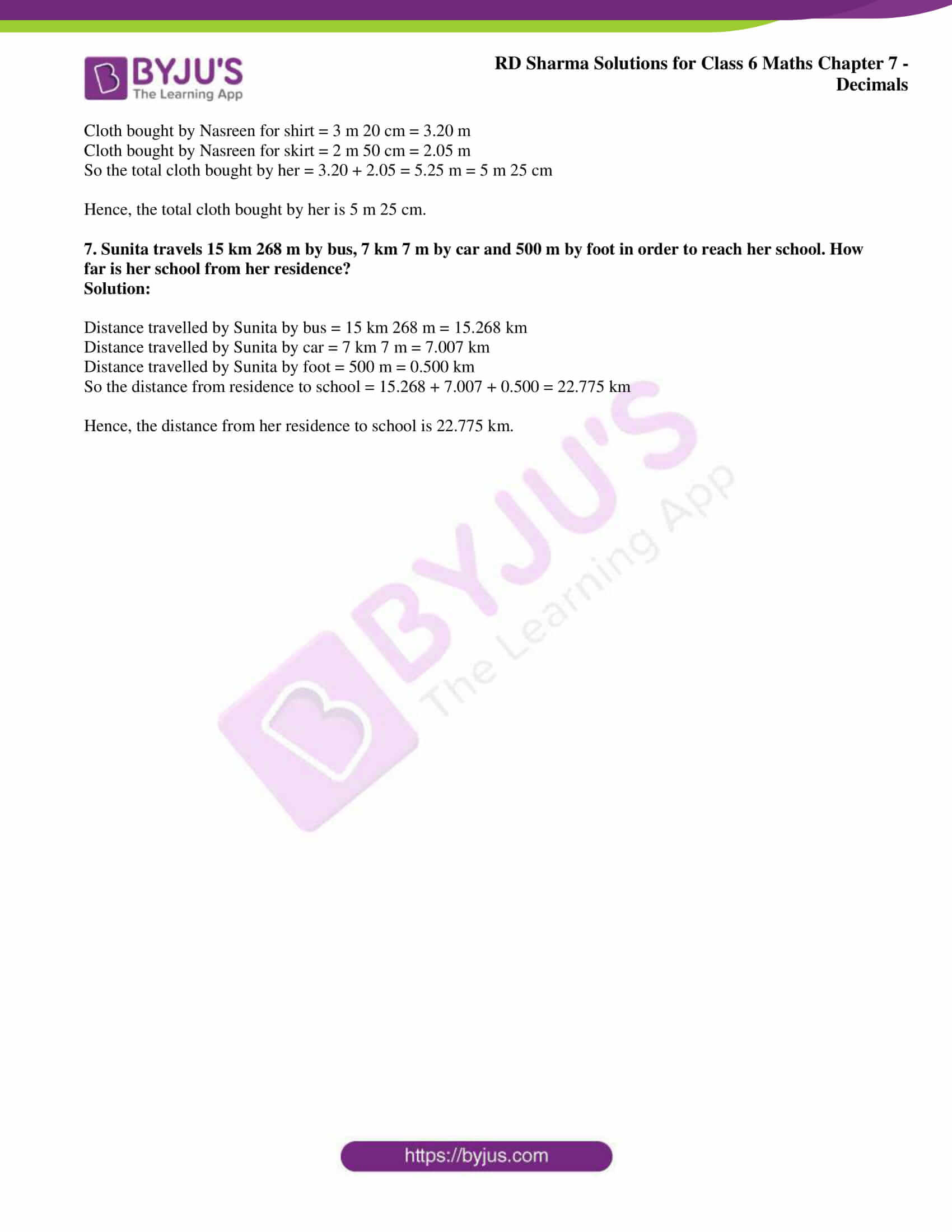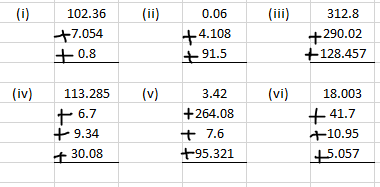# RD Sharma Solutions for Class 6 Chapter 7: Decimals Exercise 7.8

Mathematics is a major scoring subject where the fundamental concepts which are explained in Class 6 are significant for the good academic score in higher grades. RD Sharma Solutions Class 6 Exercise 7.8 covers important topics like the addition of numbers with decimals and steps followed in solving them. The students can self analyse their knowledge about the chapter using RD Sharma Solutions for Class 6 Maths Chapter 7 Decimals Exercise 7.8 PDF which are given here.

## RD Sharma Solutions for Class 6 Chapter 7: Decimals Exercise 7.8 Download PDF### Access RD Sharma Solutions for Class 6 Chapter 7: Decimals Exercise 7.8

1. Find the sum in each of the following:Solution:

(i) We know that

102.360 + 7.054 + 0.800 = 110.214

(ii) We know that

0.060 + 4.108 + 91.500 = 95.668

(iii) We know that

312.800 + 290.020 + 128.457 = 731.277

(iv) We know that

113.285 + 6.700 + 9.340 + 30.080 = 159.405

(v) We know that

3.42 + 264.08 + 7.6 + 95.321 = 370.421

(vi) We know that

18.003 + 41.700 + 10.950 + 5.057 = 75.710

(i) 41.8, 39.24, 5.01 and 62.6

(ii) 4.702, 4.2, 6.02 and 1.27

(iii) 18.03, 146.3, 0.829 and 5.324

Solution:

(i) 41.8, 39.24, 5.01 and 62.6

It can be written as

41.80 + 39.24 + 5.01 + 62.60 = 148.65

(ii) 4.702, 4.2, 6.02 and 1.27

It can be written as

4.702 + 4.200 + 6.020 + 1.270 = 16.192

(iii) 18.03, 146.3, 0.829 and 5.324

It can be written as

18.030 + 146.300 + 0.829 + 5.324 = 170.483

3. Find the sum in each of the following:

(i) 0.007 + 8.5 + 30.08

(ii) 280.69 + 25.2 + 38

(iii) 25.65 + 9.005 + 3.7

(iv) 27.076 + 0.55 + 0.004

Solution:

(i) 0.007 + 8.5 + 30.08

It can be written as

0.007 + 8.500 + 30.080 = 38.587

(ii) 280.69 + 25.2 + 38

It can be written as

280.69 + 25.20 + 38.00 = 343.89

(iii) 25.65 + 9.005 + 3.7

It can be written as

25.650 + 9.005 + 3.700 = 38.355

(iv) 27.076 + 0.55 + 0.004

It can be written as

27.076 + 0.550 + 0.004 = 27.630

4. Radhika’s mother gave her Rs 10.50 and her father gave her Rs 15.80, find the total amount given to Radhika by her parents.

Solution:

Amount given by Radhika’s mother = Rs 10.50

Amount given by Radhika’s father = Rs 15.80

So the total amount given by her parents = Rs 10.50 + Rs 15.80 = Rs 26.30

Hence, the total amount given by her parents is Rs 26.30.

5. Rahul bought 4 kg 90 g apples, 2 kg 60 g of grapes and 5 kg 300 g of mangoes. Find the weight of the fruits he bought in all.

Solution:

Weight of apples bought by Rahul = 4 kg 90 g = 4.090 kg

Weight of grapes bought by Rahul = 2 kg 60 g = 2.060 kg

Weight of mangoes bought by Rahul = 5 kg 300 g = 5.300 kg

So the weight of all the fruits = 4.090 + 2.060 + 5.300 = 11.450 kg

Hence, the weight of the fruits bought by Rahul is 11.450 kg.

6. Nasreen bought 3 m 20 cm cloth for her shirt and 2 m 5 cm cloth for skirt. Find the total cloth bought by her.

Solution:

Cloth bought by Nasreen for shirt = 3 m 20 cm = 3.20 m

Cloth bought by Nasreen for skirt = 2 m 50 cm = 2.05 m

So the total cloth bought by her = 3.20 + 2.05 = 5.25 m = 5 m 25 cm

Hence, the total cloth bought by her is 5 m 25 cm.

7. Sunita travels 15 km 268 m by bus, 7 km 7 m by car and 500 m by foot in order to reach her school. How far is her school from her residence?

Solution:

Distance travelled by Sunita by bus = 15 km 268 m = 15.268 km

Distance travelled by Sunita by car = 7 km 7 m = 7.007 km

Distance travelled by Sunita by foot = 500 m = 0.500 km

So the distance from residence to school = 15.268 + 7.007 + 0.500 = 22.775 km

Hence, the distance from her residence to school is 22.775 km.